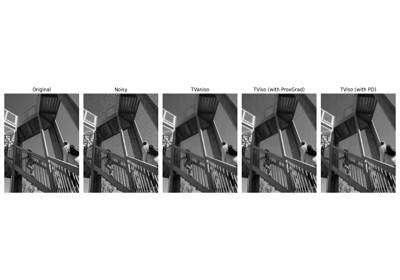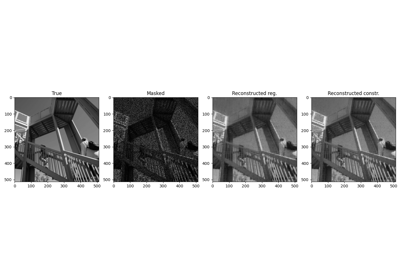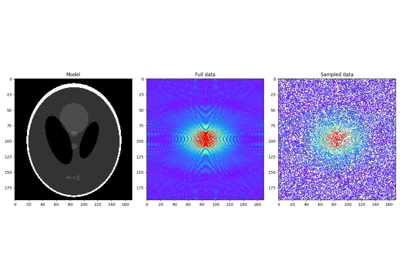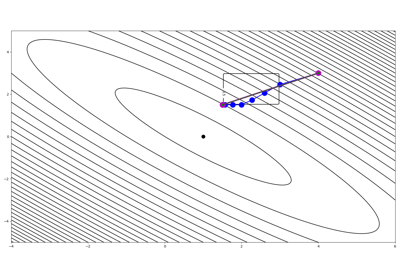pyproximal.optimization.primal.ProximalGradient(proxf, proxg, x0, tau=None, beta=0.5, epsg=1.0, niter=10, niterback=100, acceleration=None, callback=None, show=False)[source]#

Solves the following minimization problem using (Accelerated) Proximal gradient algorithm:

$\mathbf{x} = \argmin_\mathbf{x} f(\mathbf{x}) + \epsilon g(\mathbf{x})$

where $$f(\mathbf{x})$$ is a smooth convex function with a uniquely defined gradient and $$g(\mathbf{x})$$ is any convex function that has a known proximal operator.

Parameters
proxfpyproximal.ProxOperator

Proximal operator of f function (must have grad implemented)

proxgpyproximal.ProxOperator

Proximal operator of g function

x0numpy.ndarray

Initial vector

taufloat or numpy.ndarray, optional

Positive scalar weight, which should satisfy the following condition to guarantees convergence: $$\tau \in (0, 1/L]$$ where L is the Lipschitz constant of $$\nabla f$$. When tau=None, backtracking is used to adaptively estimate the best tau at each iteration. Finally note that $$\tau$$ can be chosen to be a vector when dealing with problems with multiple right-hand-sides

betafloat, optional

Backtracking parameter (must be between 0 and 1)

epsgfloat or np.ndarray, optional

Scaling factor of g function

niterint, optional

Number of iterations of iterative scheme

niterbackint, optional

Max number of iterations of backtracking

accelerationstr, optional

Acceleration (None, vandenberghe or fista)

callbackcallable, optional

Function with signature (callback(x)) to call after each iteration where x is the current model vector

showbool, optional

Display iterations log

Returns
xnumpy.ndarray

Inverted model

Notes

The Proximal point algorithm can be expressed by the following recursion:

$\begin{split}\mathbf{x}^{k+1} = \prox_{\tau^k \epsilon g}(\mathbf{y}^{k+1} - \tau^k \nabla f(\mathbf{y}^{k+1})) \\ \mathbf{y}^{k+1} = \mathbf{x}^k + \omega^k (\mathbf{x}^k - \mathbf{x}^{k-1})\end{split}$

where at each iteration $$\tau^k$$ can be estimated by back-tracking as follows:

\begin{split}\begin{aligned} &\tau = \tau^{k-1} &\\ &repeat \; \mathbf{z} = \prox_{\tau \epsilon g}(\mathbf{x}^k - \tau \nabla f(\mathbf{x}^k)), \tau = \beta \tau \quad if \; f(\mathbf{z}) \leq \tilde{f}_\tau(\mathbf{z}, \mathbf{x}^k) \\ &\tau^k = \tau, \quad \mathbf{x}^{k+1} = \mathbf{z} &\\ \end{aligned}\end{split}

where $$\tilde{f}_\tau(\mathbf{x}, \mathbf{y}) = f(\mathbf{y}) + \nabla f(\mathbf{y})^T (\mathbf{x} - \mathbf{y}) + 1/(2\tau)||\mathbf{x} - \mathbf{y}||_2^2$$.

Different accelerations are provided:

• acceleration=None: $$\omega^k = 0$$;

• acceleration=vandenberghe : $$\omega^k = k / (k + 3)$$ for

• acceleration=fista: $$\omega^k = (t_{k-1}-1)/t_k$$ for where $$t_k = (1 + \sqrt{1+4t_{k-1}^{2}}) / 2$$ 

1

Vandenberghe, L., “Fast proximal gradient methods”, 2010.

2

Beck, A., and Teboulle, M. “A Fast Iterative Shrinkage-Thresholding Algorithm for Linear Inverse Problems”, SIAM Journal on Imaging Sciences, vol. 2, pp. 183-202. 2009.

## Examples using pyproximal.optimization.primal.ProximalGradient#Denoising

DenoisingLow-Rank completion via SVD

Low-Rank completion via SVDNonlinear inversion with box constraints

Nonlinear inversion with box constraintsPlug and Play Priors

Plug and Play Priors